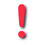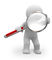# Introduction and notation(If you are experiencing problems with the display of the mathematical formula, you can either try to use another browser, or use this link which should work smoothly: http://popix.lixoft.net)

## Different representations of the same model

The description of a model requires variables such as observations $(y_i)$, individual parameters $(\psi_i)$, population parameters $\theta$, covariates $(c_i)$, etc.

Tasks to be performed (estimation, simulation, likelihood calculation, etc.) involve these variables. Algorithms used to perform these tasks can use different parameterizations, i.e., different mathematical representations of the same model. We will see that depending on the task, some mathematical representations are more suitable than others.

There exists for a modeler a natural parametrization involving a vector of individual parameters $\psi_i$ which have a physical or biological meaning (rate, volume, bioavailability, etc.). We will denote by $\psi$-representation the mathematical representation of the model which uses $\psi_i$:

 $$\pyipsii(y_i , \psi_i ; \theta) = \pcyipsii(y_i | \psi_i)\ppsii( \psi_i ; \theta, c_i).$$ (1)

When there exists a transformation $h: \Rset^d \to \Rset^d$ such that $\phi_i=h(\psi_i)$ is a Gaussian vector, we can use equivalently the $\phi$-representation which involves the transformed parameters (log-rate, log-volume, logit-bioavailability, etc.) and now represents the joint distribution of $y_i$ and $\phi_i$:

 $$\pyiphii(y_i , \phi_i ; \theta, c_i) = \pcyiphii(y_i | \phi_i)\pphii( \phi_i ; \theta, c_i),$$ (2)

where $\phi_i =h(\psi_i) \sim {\cal N}( \mu(\beta,c_i) , \Omega)$ and $\theta=(\beta,\Omega)$.

There is yet another mathematical representation which uses the vector of random effects $\eta_i$ to represent the individual parameters model:

$$\begin{eqnarray} \phi_i &=& \mu(\beta,c_i) + \eta_i , \end{eqnarray}$$

where $\eta_i \sim {\cal N}( 0 , \Omega)$. This $\eta$-representation leads to the joint distribution of $y_i$ and $\eta_i$:

 $$\pyietai(y_i , \eta_i ; \theta, c_i) = \pcyietai(y_i | \eta_i;\beta,c_i)\petai( \eta_i ; \Omega).$$ (3)

We can see that the fixed effects $\beta$ now appear in the conditional distribution of the observations. This will have a strong impact on tasks such as estimation of population parameters since a sufficient statistic for estimating $\beta$ derived from this representation will be a function of the observations $\by$, as opposed to the other representations, where the sufficient statistic is a function of the individual parameters $\bpsi$ (or equivalently, $\bphi$).

In the $\psi$-representation (1), if the model $\ppsii( \psi_i ; \theta, c_i)$ is not a regular statistical model (some components of $\psi_i$ may have no variability, or more generally $\Omega$ may not be positive definite), no sufficient statistic $S(\psi_i)$ for estimating $\theta$ exists. Thus, estimation algorithms will not use representation (1) in these cases, but another decomposition into regular statistical models.Some examples

1. Consider the following model for continuous data with a constant error model:
2. $$\begin{eqnarray} y_{ij} &\sim& {\cal N}(f(t_{ij},\phi_i) ,a_i^2) \\ \phi_i &\sim& {\cal N}(\beta, \Omega) \\ a_i &\sim& p_a(\, \cdot \, ; \theta_a) . \end{eqnarray}$$

Here, the variance of the residual error is a random variable. The vector of individual parameters is $(\phi_i, a_i)$ and the vector of population parameters is $\theta=(\beta,\Omega,\theta_a)$. Assuming that $\Omega$ is positive definite, the joint model of $y_i$, $\phi_i$ and $a_i$ can be decomposed as a product of three regular models:

$$\pyiphii(y_i , \phi_i, a_i ; \theta) = \pcyiphii(y_i | \phi_i ,a_i)\pphii( \phi_i ; \beta, \Omega)\pmacro(a_i ; \theta_a).$$

3. Assume instead that the variance of the residual error is fixed for the whole population:
4. $$\begin{eqnarray} y_{ij} &\sim& {\cal N}(f(t_{ij},\phi_i) ,a^2) . \end{eqnarray}$$

The vector of population parameters is now $\theta=(\beta,\Omega,a)$ and the joint model of $y_i$ and $\phi_i$ can be decomposed as

$$\pyiphii(y_i , \phi_i ; \theta) = \pcyiphii(y_i | \phi_i ; a)\pphii( \phi_i ; \beta, \Omega).$$

5. Suppose that some components of $\phi_i$ have no inter-individual variability. More precisely, let $\phi_i=(\phi_i^{(1)} \phi_i^{(0)})$ and $\beta=(\beta_1,\beta_0)$, such that
6. $$\begin{eqnarray} \phi_i^{(1)} &\sim& {\cal N}(\beta_1, \Omega_1) \\ \phi_i^{(0)} &=& \beta_0 , \end{eqnarray}$$

and $\Omega_1$ is positive definite. Here, $\theta=(\beta_1,\beta_0,\Omega_1,a)$ and

$$\pyiphii(y_i , \phi_i^{(1)} ; \theta) = \pcyiphii(y_i | \phi_i^{(1)} ; \beta_0, a)\pphii( \phi_i^{(1)} ; \beta_1, \Omega_1).$$

7. Assume instead that $\phi_i = (\phi_{i,1}, \phi_{i,2})$, where
8. $$\begin{eqnarray} \phi_{i,1} &=& \beta_1 + \omega_1\eta_i \\ \phi_{i,2} &=& \beta_2 + \omega_2\eta_i , \end{eqnarray}$$

and $\eta_i \sim {\cal N}(0,1)$. Here, the useful model is the joint distribution of $y_i$ and $\eta_i$. We can use for instance the following $\eta$-representation:

$$\pyietai(y_i , \eta_i ; \theta) = \pcyietai(y_i | \eta_i ;\theta)\petai( \eta_i),$$

where $\theta= (\beta_1,\beta_2, \omega_1,\omega_2,a)$.

## Some notation

We assume that the set of population parameters $\theta$ takes its values in $\Theta$, an open subset of $\Rset^m$.

Let $f : \Theta \to \Rset$ be a twice differentiable function of $\theta$. We will denote $\Dt{f(\theta)} = (\partial f(\theta)/\partial \theta_j, 1 \leq j \leq m)$ the gradient of $f$ (i.e., the vector of partial derivatives of $f$) and $\DDt{f(\theta)} = (\partial^2 f(\theta)/\partial \theta_j\partial \theta_k, 1 \leq j,k \leq m)$ the Hessian of $f$ (i.e., the square matrix of second-order partial derivatives of $f$).